# Simple melody with buzzer

Now it’s time to make some noise🎶.

In this tutorial, you’ll work with a new component - buzzer. You'll make it play a scale. Besides, you will adjust LED brightness with PWM signal. Let’s figure it out together.

Learning goals

• Clarify the working principle of the PWM signal.
• Know two different types of buzzers.
• Understand how a buzzer produces sound.
• Learn to control LED brightness with PWM output.

## 🔸Background​

### What is PWM?​

In the previous projects, you tried the digital output to turn on and off an LED. But how can you make the LED dimmer or brighter? Well, the pulse width modulation (PWM) can deal with it. Let's first learn about the PWM.

As you know, a digital signal always outputs high or low level. The PWM signal is still a square wave signal with two states: on (when the signal is high) and off (when the signal is low). The on-time of the signal is called pulse width. So as its name suggests, PWM refers to the method of changing the pulse width, that is, the proportion of on-time to control the output. The signal would change extremely quickly between on and off. It's so fast that your eyes can't notice the change. In this way, you seem to get an average voltage between 0V and 3.3V.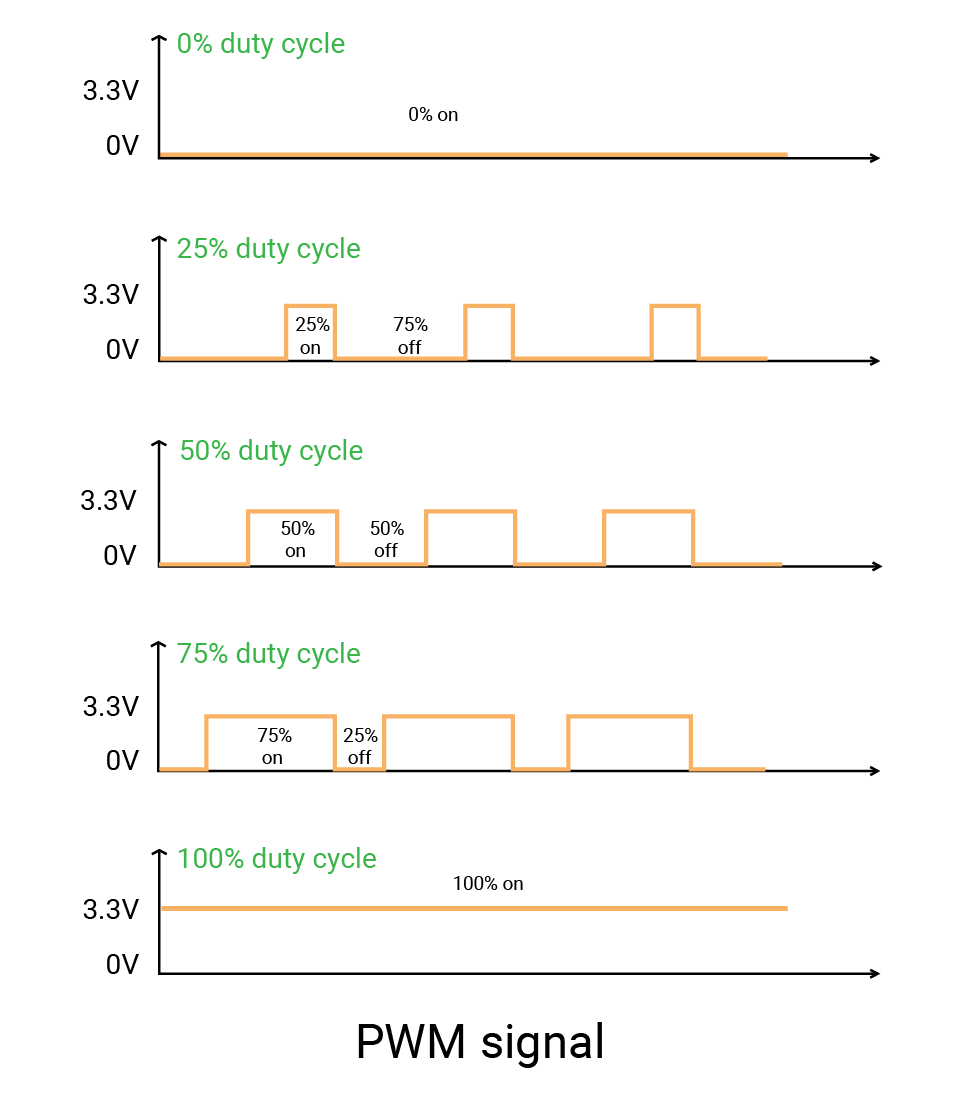Here are some more concepts about PWM:

• The duty cycle describes the percentage of the on-time in a period:

• 0 means the signal is always low.
• 1 means it is always high.
• A signal with a duty cycle of 0.5 should be high for 50% of the time and low for 50% of the time. And the average voltage is 1.65V.
• So a signal with any duty cycle between 0 and 1 can simulate a voltage between 0 and 3.3V.
• One period of the signal consists of on-time and off-time. It describes the time that a cycle lasts. The frequency is the inverse of the period. It tells the count of cycles in one second. Take 1000Hz for example, the signal has 1000 cycles per second, and the period is 1ms.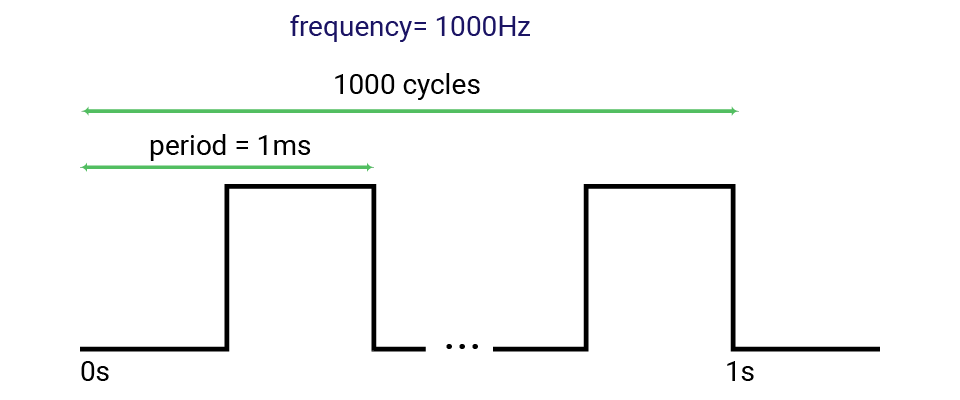PWM is useful in many situations: adjusting LED brightness, controlling a servo, or making a buzzer produce sound. You may adjust the duty cycle or the frequency of signals according to your projects. In this tutorial, a buzzer needs different frequencies to generate different notes, while an LED want varying duty cycles to show brightness change.

## 🔸New component​

### Buzzer​

The buzzer is a kind of audio device to produce beeping and sharp sounds. It is widely used for notification and confirmation. You may hear it from alarms, toys, or when pressing buttons.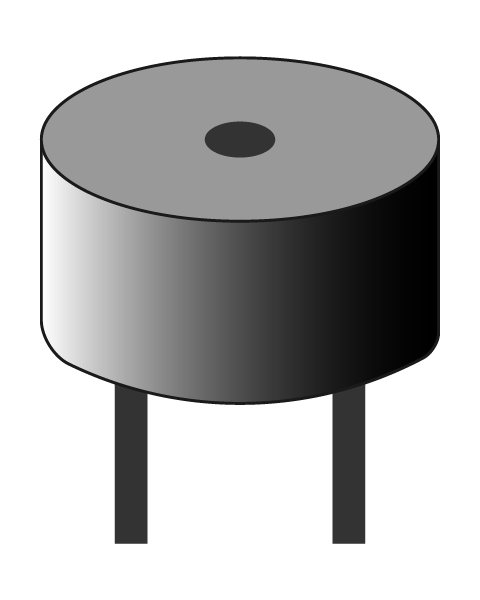Symbol: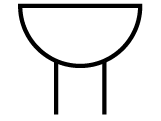How does it emit the sound? It's due to the diaphragm inside it. As you apply to a buzzer a current that changes with time, the internal diaphragm will vibrate back and forth quickly. The vibration of the diaphragm thus vibrates the surrounding air, hence producing a sound. When there is no current, the diaphragm will go back to its original position, the sound thus stops.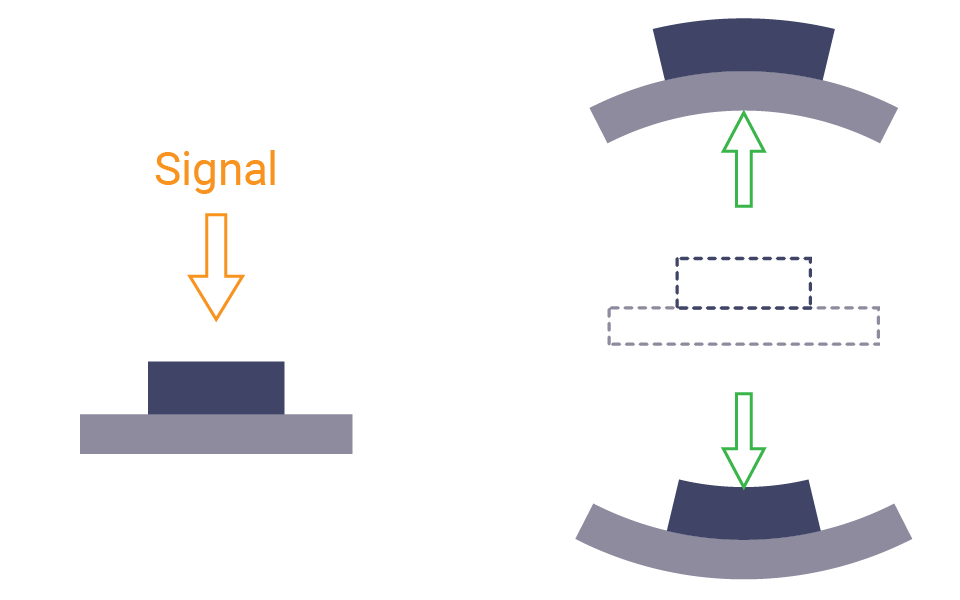There are two kinds of buzzers: active buzzer and passive buzzer. They needs different types of current.

• The active buzzer contains an internal circuit that can create varying voltage. So once you power this kind of buzzer, it will make a sound automatically. But it can only produce one constant sound since the wave generated by the internal circuit is determined. What's more, the active buzzer is polarised and needs to connect in the right direction.
• The passive buzzer needs a PWM signal to drive it. The pitch of a sound depends on the frequency of the signal. Higher frequency would move the diaphragm faster and lead to a higher pitch. And the one in the kit is a passive buzzer.

They looks alike. To distinguish them, you can connect them to power. If it sounds, it is an active buzzer.

## 🔸New concept​

### Pitch​

You must be familiar with do, re, mi... They correspond respectively to the note C, D, E, F, G, A, B. Please note the notes start from C but aren't in alphabetical order.

The pitch of each note is decided by the frequency. High pitch corresponds to high frequency, low pitch corresponds to low frequency. The following table gives the frequency of each note. When it comes to Middle C (octave 4), which is the first note to find when you start to learn the piano, the frequency is about 262 Hz.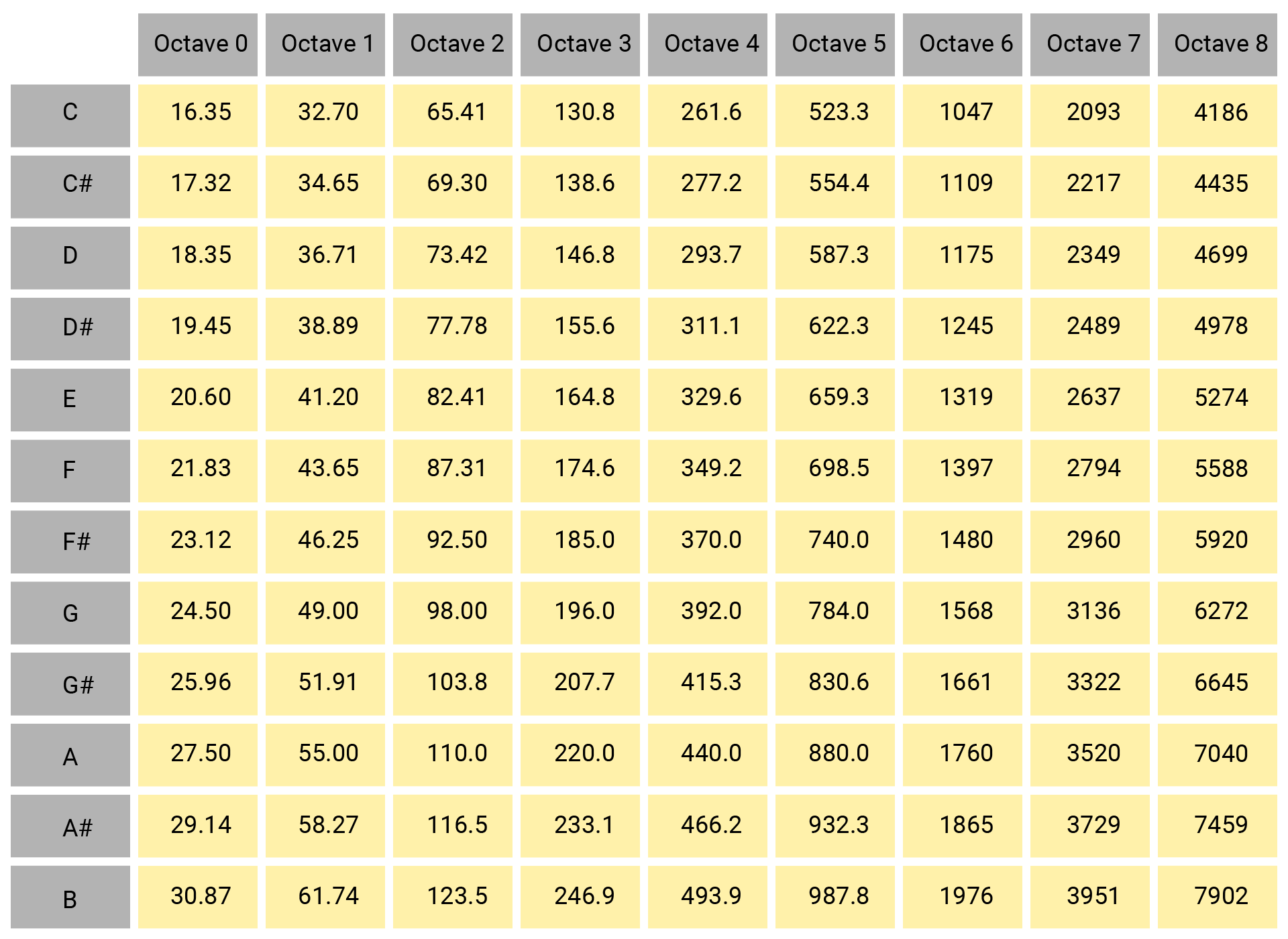A piano keyboard would be helpful to understand the concept. All keys on the keyboard has a corresponding pitch. They are divided into several octaves: 0 to 7.

Let's look the octave 4 in the middle. The white keys corresponds to the notes C, D, E, F, G, A, B. And the note C in this octave is the middle C. The other octave are similar, but will be higher or lower in pitch according to the frequencies.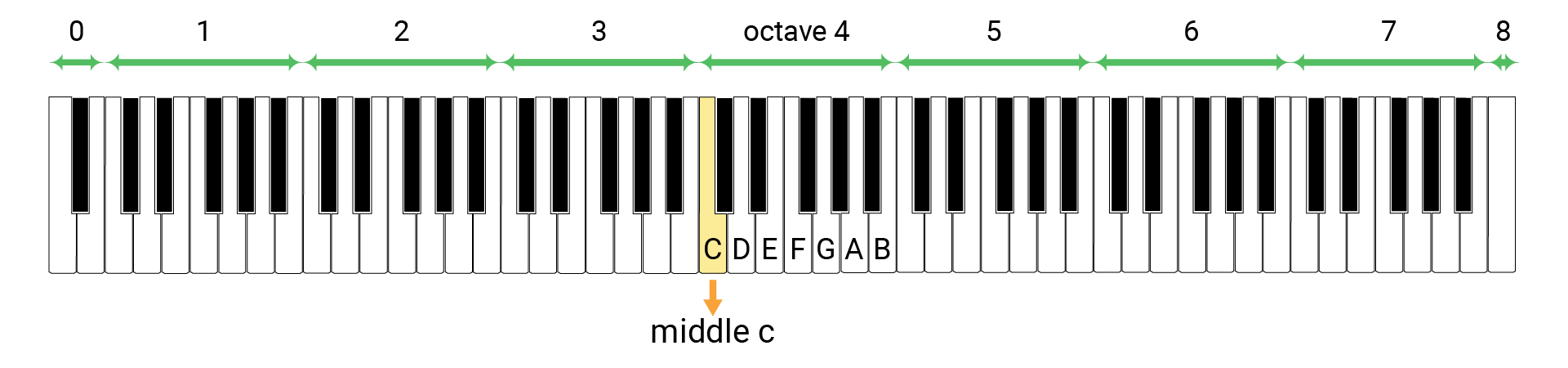So to make the buzzer play a piece of melody, you need to find the frequency and set a proper duration for each note according to the score.

## 🔸Circuit - buzzer module​

The buzzer is connected to the pin PWM5A.

The PWM pins are marked with a tilde (~) on the silkscreen of your board. Most of the pins on your SwiftIO Feather board are multifunctional. A pin may be used as digital pins and PWM pins. You can refer to this pinout to know the functionalities of each pin.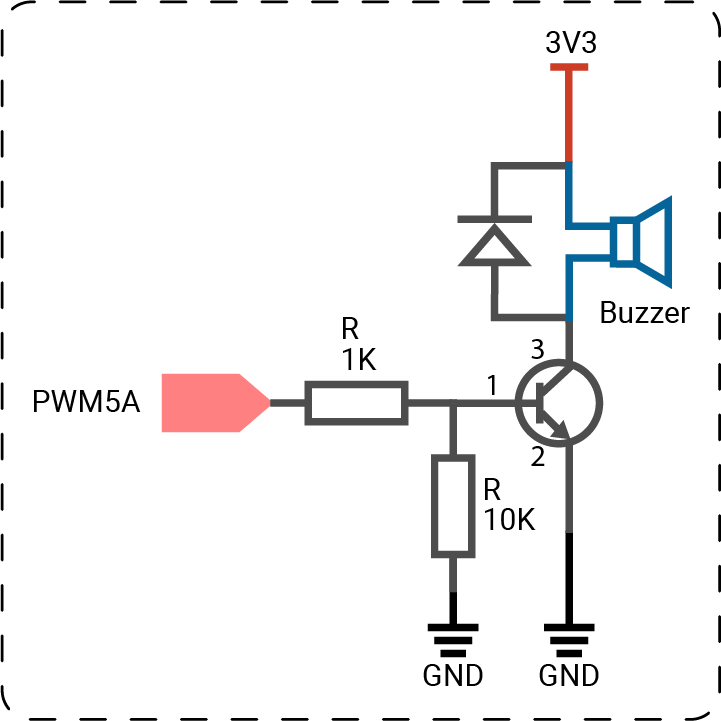##### note

The circuits above are simplified for your reference.

## 🔸Preparation​

Class

`PWMOut` - this class allows you to set the frequency and duty cycle of PWM output from the board.

MethodExplanation
`init(_:frequency:`
`dutycycle:)`
Initialize a PWM output pin.
- `id`: id of the pin.
- `frequency`: frequency of the PWM signal, 1000Hz by default.
- `dutycycle`: a float representing duty cycle of the PWM, 0 by default.
`set(frequency:`
`dutycycle:)`
Set the frequency and duty cycle of the PWM signal.
`setDutycycle(_:)`Set the duty cycle of the PWM signal.
`suspend()`Suspend the PWM signal.

## 🔸Projects​

### 1. Musical scale​

In this project, the buzzer will play the musical scale.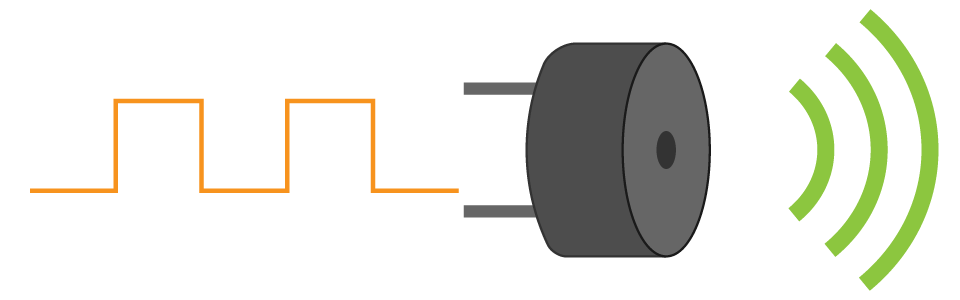Example code

``// Import the SwiftIO library to set the input and output, and the MadBoard to use the pin id.import SwiftIOimport MadBoard// Initialize a PWM pin for the buzzer.let buzzer = PWMOut(Id.PWM5A)// Declare a constant to store an array of frequencies. // Consult the pitch-frequency chart above and list all the necessary frequencies in order in the array.let frequencies = [262, 294, 330, 349, 392, 440, 494, 523]// Use the for-in loop to iterate through each frequency.// Set the frequency of the PWM signal to generate sounds. Each note will last 1s.for frequency in frequencies {    buzzer.set(frequency: frequency, dutycycle: 0.5)    sleep(ms: 1000)}// Stop the buzzer sound.buzzer.suspend()while true {    sleep(ms: 9999)}``

Code analysis

``let buzzer = PWMOut(Id.PWM5A)``

The id of PWM pins are a little different. You will see A or B in the end. Actually, the PWM pins are grouped in pairs, like PWM2A and PWM2B. The two pins in a group share the same frequency. So if you need two PWM pins with different frequencies, you should use the pins in different groups, like PWM0A and PWM1A.

``let frequencies = [262, 294, 330, 349, 392, 440, 494, 523]``

Here you create an array to store the frequencies of each note from C (octave 4) to C (octave 5).

The `frequencies` is a constant, so the array cannot be changed later. If you want to change the array, you need to change `let` to `var` to make it a variable.

``for frequency in frequencies {}``

Iterate through each value in the array `frequencies` using for-in loop.

``buzzer.set(frequency: frequency, dutycycle: 0.5)``

You are going to set the frequencies, so the method `set(frequency:dutycycle:)` is more suitable in this case.

The frequency corresponds to the values in the array. And the duty cycle could be any float number between 0.0 and 1.0.

``buzzer.suspend()``

It will suspend the output, thus stop the sound from the buzzer. If you remove this statement, the last note will last forever.

### 2. Breathing LED​

After the musical scale with the buzzer, let’s go back to the LED again.

The PWM signal can not only drive a buzzer, but also change LED brightness. By applying PWM output, the LED changes between on and off quickly. You could not notice this change, so it seems the LED becomes brighter and dimmer. BTW, your monitor may also use this method to control the backlight. A bigger duty cycle will make the LED brighter, since the time for high level is much longer.

So let’s try a breathing LED! You may notice it when a new message comes on your phones. The LED changes from the darkest to the brightest, from brightest to darkest smoothly. It looks like it is breathing.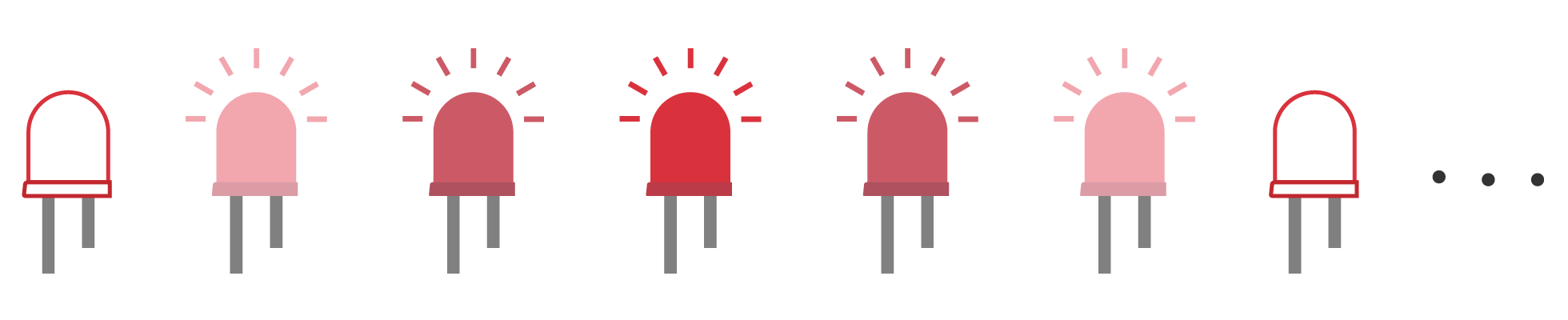Example code

``// Import the SwiftIO library to set the input and output and the MadBoard to use the pin id.import SwiftIOimport MadBoard// Initialize a PWM output pin for the LED.let led = PWMOut(Id.PWM4A)// Set the PWM output to control the LED. // The frequency is set to 1000Hz or can be other value. // The duty cycle is 0 in the beginning so LED keeps turning off.led.set(frequency: 1000, dutycycle: 0)// Store the maximum and minimum values of the duty cycle to two constants. let maxDutycycle: Float = 1.0let minDutycycle: Float = 0.0// Set the change of duty cycle for each action.let stepDutycycle: Float = 0.01// Create a variable to store the varying duty cycle.var dutycycle: Float = 0.0// A condition used to decide whether to increase or decrease the duty cycle. var upDirection = truewhile true {    // Output a PWM signal with the specified duty cycle to control LED brightness.    led.setDutycycle(dutycycle)    // Keep each brightness last for 10ms, or you may not see the changes.    sleep(ms: 10)    // Increase or decrease the duty cycle within its range according to the value of upDirection.    if upDirection {        dutycycle += stepDutycycle        if dutycycle >= maxDutycycle {            upDirection = false        }    } else {        dutycycle -= stepDutycycle        if dutycycle <= minDutycycle {            upDirection = true        }    }}``

Code analysis

``let maxDutycycle: Float = 1.0let minDutycycle: Float = 0.0let stepDutycycle: Float = 0.01``

There are some built-in data types in the Swift language, like Int (integer), Float and Double (decimal number), Bool (true and false), etc. Each type corresponds to different values type and value range. If the values in your program don't match or exceed the default range, the compilation will fail.

Besides, if you don’t explicitly declare the data type, Swift will use type inference to decide the type. For example, if the `minDutycycle` is written as 0, Swift would judge it as an Int. When dealing with decimal numbers 0.0, it could be either float or double. The default choice of Swift is double. However, the duty cycle used in `PWMOut` class is `Float`. Therefore, the values above need to be declared as `Float`.

The LED brightness depends on the duty cycle of the PWM signal. The duty cycle is always between 0 and 1. So the maximum and minimum values are stored in two constants for later comparison.

The constant `stepDutycycle` is the change of the duty cycle. You'll increase and decrease the duty cycle by the value repeatedly. Make sure `stepDutycycle` is not too big so the brightness changes smoothly.

``var dutycycle: Float = 0.0``

A variable, like a constant, is a container for a value but can be changed with other values of the same type. In this case, the duty cycle will always change, so it is stored as a variable instead of a constant.

``var upDirection = true``

This variable is used as a condition to decide whether the LED will become brighter or dimmer. In the beginning, it's true so the LED will become brighter at first.

``led.setDutycycle(dutycycle)``

Set the duty cycle of PWM output. The LED brightness will change according to its value.

``sleep(ms: 10)``

It makes each brightness last for 10ms. If you remove this statement, the LED changes so fast that you cannot notice the brightness change.

The duty cycle is 0 - 1, and the change is 0.01 each time. So one loop will last 100 times. Then let’s suppose the LED changes from off to full brightness in 1 second, and you will get the duration for each state - 10ms.

``if upDirection {    ...} else {    ...}``

This conditional statement will decide how the duty cycle will change according to `upDirection`.

• If it's true, the duty cycle will increase by the value of `stepDutycle`. Once its value exceeds the maximum, `upDirection` will be false.

• If it’s false, the duty cycle will decrease. When it is below its minimum, `upDirection` becomes `true` again.

``dutycycle += stepDutycycle``

+= combines the addition and assignment. It equals `dutycycle = dutycycle + stepDutycycle`.

And -= means `dutycycle = dutycycle - stepDutycycle`.

``if dutycycle >= maxDutycycle {    upDirection = false}``

This statement is to know whether the duty cycle exceeds its range. If so, `upDirection` is changed to `false`. In next loop, the duty cycle will begin to decrease by `stepDutycycle`.

### 3. LED brightness control​

You'll adjust the brightness of the LED using two buttons: one is reserved to increase the brightness, the other is to reduce the brightness.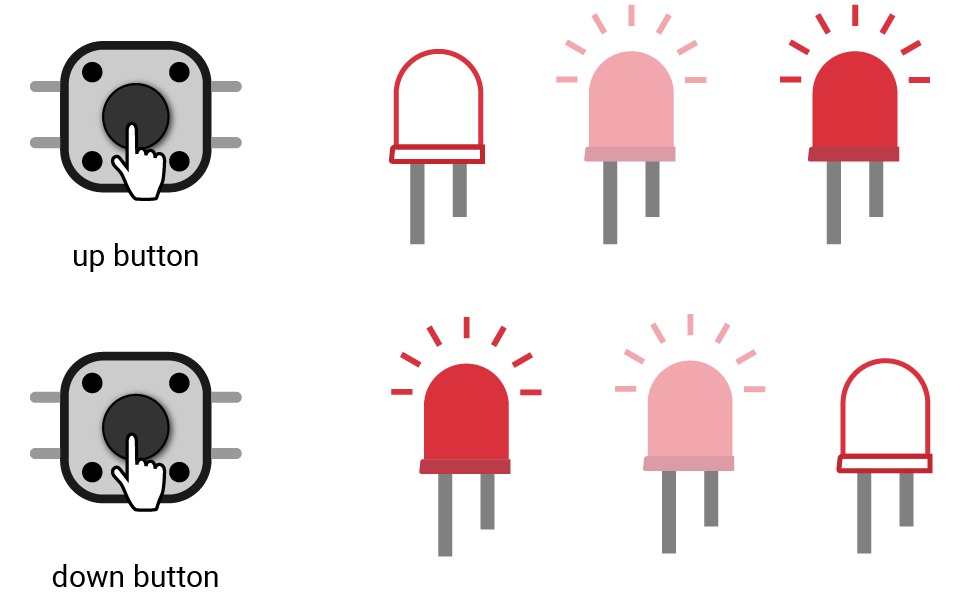Example code

``// Import two necessary libraries.import SwiftIOimport MadBoard// Initialize the PWM pin.let led = PWMOut(Id.PWM4A)// Set the frequency of the PWM signal and set the duty cycle to 0 to keep the LED off.led.set(frequency: 1000, dutycycle: 0)// Store the max and min values of duty cycle to two constants. let maxDutycycle: Float = 1.0let minDutycycle: Float = 0.0// The variation of duty cycle per button press.let stepDutycycle: Float = 0.1// Create a variable to store the value of duty cycle.var dutycycle: Float = 0.0// Initialize the digital pins. downButton is to dim the LED and the upButton is to brighten the LED.let downButton = DigitalIn(Id.D1)let upButton = DigitalIn(Id.D21)// Each time this button is pressed, the LED will dim a little until it reaches the minimum brightness.downButton.setInterrupt(.rising) {    dutycycle -= stepDutycycle    dutycycle = max(dutycycle, minDutycycle)    led.setDutycycle(dutycycle)}// Once this button is pressed, the LED becomes brighter until it reaches the maximum brightness.upButton.setInterrupt(.rising) {    dutycycle += stepDutycycle    dutycycle = min(dutycycle, maxDutycycle)    led.setDutycycle(dutycycle)}// Keep the board sleeping when the button is not pressed.while true {    sleep(ms: 1000)}``

Code analysis

``downButton.setInterrupt(.rising) {    dutycycle -= stepDutycycle    dutycycle = max(dutycycle, minDutycycle)    led.setDutycycle(dutycycle)}``

The two button `upButton` and `downButton` works similarly. Take `downButton` for example.

You'll use the interrupt mechanism to detect if the button is pressed.

There are three statements for the interrupt. Each of them can be executed in a very short time and thus can be used as ISR.

`max()` is used to get the biggest one between the values. In this way, even if the result of the calculation is smaller than its minimum (0.0), the duty cycle is still 0.0 to keep the LED at the minimum brightness.